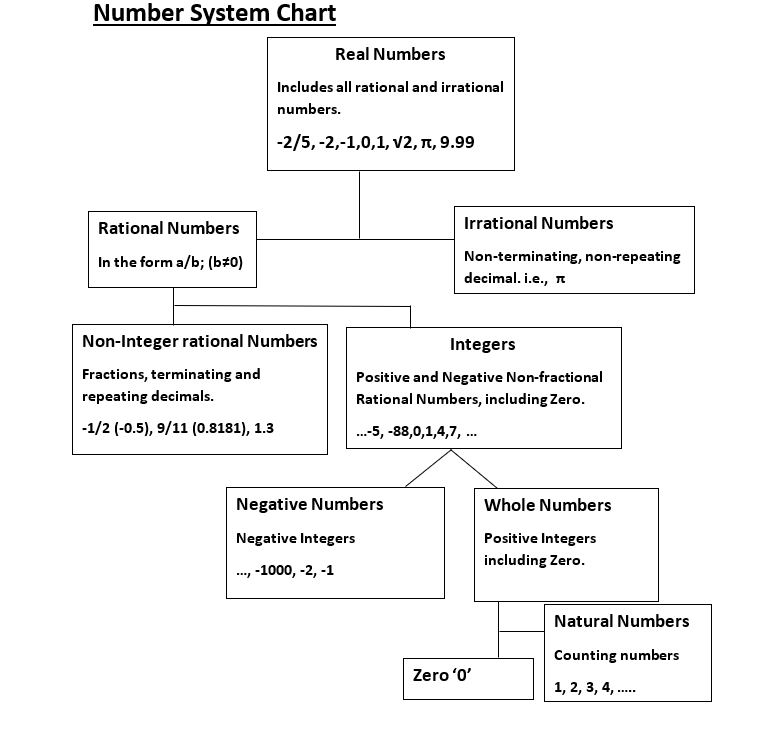Number System Chart
14 May 2020

Introduction

The number system is the basis of entire basic numeracy. Most of its content is already been studied at school level. Almost one-fifth of every competitive examination questions are from this chapter, so it is expected from the students to prepare this chapter well.

#### Number System ChartNow, let us look at the type of numbers one by one.

## Did You Know

The number 2 is the only even prime number.

There are 25 prime numbers between 1 and 100.

The two consecutive numbers are always co-prime numbers.